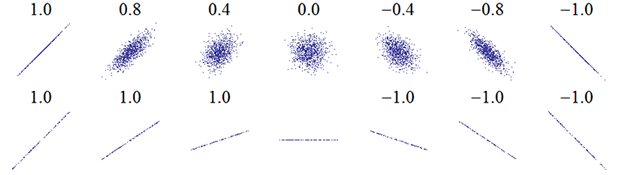We use the Correlation coefficient to quantify the strength and direction of a relationship between two variables. $$e.g.$$, think about height and weight, or hours of sleep and irritability.

$$\bullet$$ The Pearson product-moment correlation coefficient is scale free and it ranges between -1 and 1.
$$\bullet$$ It is typically denoted by $$r$$, for sample data or by $$\rho$$ (the greek symbol Rho), to indicate the population value.
$$\bullet$$ You have probably examined XY scatterplots to visualize this type of bivariate relationship, and have begun to evaluate the 2 dimensional attributes of the scattercloud to gain a sense of direction and strength of the relationship.

$$\bullet$$ Often, introductory textbooks show a figure like the following which depicts a series of XY scatterplots reflecting correlation patterns of differing size and sign. This one is the Wikipedia illustration.$$\bullet$$ A correlation of -1 means that the X and Y variables have a perfect negative relationship and the data points fit a straight line with a negative slope.
$$\bullet$$ Similarly, a correlation of +1 means that X and Y have a perfect positive relationship and fall on a line with positive slope.
$$\bullet$$ If X and Y variables have no relationship, the correlation is 0, and the array of points in the scatterplot have no pattern/orientation.

#### The user is advised to work through each tab, in order.

Shiny app by Bruce Dudek

##### Simulation No. 1. Visualize direction of relationship with Quadrants.

$$\bullet$$ If we randomly sample Y and X variables from a bivariate normal population/distribution,we can draw an XY scatterplot of the N data points.
$$\bullet$$ XY Relationships can be explored here by changing parameters and by resampling.
$$\bullet$$ Note that even though you may fix $$\rho$$ at one value, the $${r_{xy}}$$ values will change with resampling. This reflects the sampling 'noise' of the statistic. Try to see how increasing sample size influences this variation in r from sample to sample

##### Simulation No. 2. Visualizing the Cross-Product computation.

$$\bullet$$ Rho ($$\rho_{xy}$$), the population correlation is defined as $$\frac{{{\sigma _{xy}}}}{{{\sigma _x}{\sigma _y}}}$$. The numerator is the Covariance of X and Y, and the denominator is the product of the two standard deviations.
$$\bullet$$ With sample data $${r_{xy}}$$ is computed as $$\frac{{Co{v_{xy}}}}{{{s_x}{s_y}}}$$. The covariance term in the numerator is the central statistic in determining the relationship between the X and Y variables.
$$\bullet$$ The $$Co{v_{xy}}$$ is calculated with sample data as $$\frac{{\sum\limits_{i = 1}^n {({X_i} - \bar X)({Y_i} - \bar Y)} }}{{n - 1}}$$. The numerator of the covariance statistic is call the Sum of Cross-Products (SP).
$$\bullet$$ Each pair of X and Y values contribute this deviation product and each is called a Cross-Product.
$$\bullet$$ The simulation here enables visualization of one of these cross-products and enables the viewer to relate it to the quadrant-location perspective seen in simulation 3.

##### Simulation No. 3. Visualizing the Cross-Product as a Rectangle.

$$\bullet$$ We saw above, that the covariance statistc is central to the concept of a correlation. It is computed with the following expression: $$\frac{{\sum\limits_{i = 1}^n {({X_i} - \bar X)({Y_i} - \bar Y)} }}{{n - 1}}$$.
$$\bullet$$ The numerator of the covariance was defined as the Sum of Cross Products, $$\sum\limits_{i = 1}^n {({X_i} - \bar X)({Y_i} - \bar Y)}$$, often abbreviated as SP.
$$\bullet$$ The SP is a summation of a multiplication operation done on each case and we have defined this multiplication as a Cross Product: $$({X_i} - \bar X)({Y_i} - \bar Y)$$.
$$\bullet$$We examined these deviations in Simulation 2 and alluded to the area that they circumscribe as a rectangle
$$\bullet$$ The simulation here enables explicit visualization of one of these cross-products as a rectangle.
$$\bullet$$ Since the area of a rectangle is the product of two adjacent sides, the value of a Cross Product can be seen as the area of a rectangle.

$$\bullet$$ Simulation No. 4. Visualize All Cross-Products as Rectangles.
$$\bullet$$ In this simulation positive and negative Cross Products can be visualized.
$$\bullet$$ Since the Sum of Cross Products ($$\sum\limits_{i = 1}^n {({X_i} - \bar X)({Y_i} - \bar Y)}$$) is the sum of all of these areas, it can be visualized as the aggregate of the positively signed rectangle areas and the negatively signed rectangle areas. Consistent with the sign of the correlation coefficient, this sum can be either negative or positive.
$$\bullet$$ Manipulation of the scaling aspects by changing scale (SD's) can change the SP dramatically, even when the correlation is held constant.

## Tools for Statistics Instruction using R and Shiny

Author: Bruce Dudek at the University at Albany, with assistance from Jason Bryer (Excelsior College)

The simulation approach in this application simulates samples drawn from a bivariate normal distribution, where the means, sd's, rho, and n are specified by the user. The randomly drawn sample results are displayed in XY scatterplots along with unique approaches to visualizing the connection between computations and the strength/sign of the correlation.

In particular, some of the simulations emphasize a visualization of the construction of the covariance statistic.

Built using Shiny by Rstudio and R, the Statistical Programming Language.

The correlation/covariance simulation uses the rmvnorm function in the mvtnorm package in R.

Ver 1.2, May 23, 2018